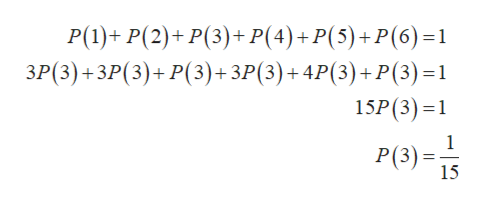# Suppose die is weighted such that the probability of rolling a three is the same as rolling a six, the probability of rolling a one, two, or four is 3 times that of a six, and the probability of rolling a five is 4 times that of rolling a three. Find the probability ofRolling a onerolling a tworolling a threerolling a four

Question

Suppose die is weighted such that the probability of rolling a three is the same as rolling a six, the probability of rolling a one, two, or four is 3 times that of a six, and the probability of rolling a five is 4 times that of rolling a three. Find the probability of

Rolling a one

rolling a two

rolling a three

rolling a four

check_circle

Step 1

Suppose die is weighted such that the probability of rolling a three is the same as rolling a six, the probability of rolling a one, two, or four is 3 times that of a six, and the probability of rolling a five is 4 times that of rolling a three. Then

Step 2

The total probability of the events of rolling one, rolling2, ...help_outlineImage TranscriptioncloseР(1)+ P(2)+ P(3)+ P(4)+P(5)+P(6)-1 ЗР(3) + ЗР (3)+ Р(3)+ ЗР(3)+4P(3)+ P(3) -1 15P(3)1 1 Р(3) -3; 15 fullscreen

### Want to see the full answer?

See Solution

#### Want to see this answer and more?

Solutions are written by subject experts who are available 24/7. Questions are typically answered within 1 hour.*

See Solution
*Response times may vary by subject and question.
Tagged in

### Math